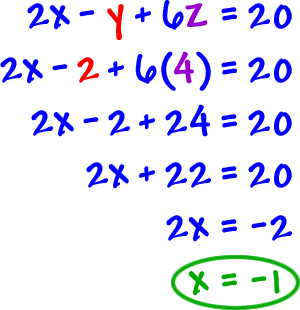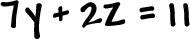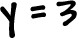Now, we have z = 4 and y = 2 ...  Stick them both into the top equation and we'll get x!So, x = -1, y = 2 and z = 4.  Since this is a point in space, we write the answer as a 3-D coordinate:

(-124)

Yeah, that was just the END of one of these problems!  Really, it just looks like a lot because of all my explaining.

TRY IT:

Just work your way back up: